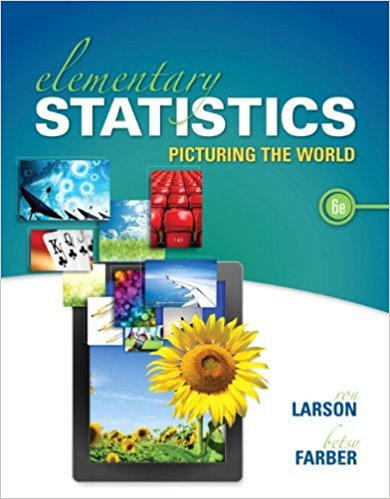×
Get Full Access to Elementary Statistics: Picturing The World - 6 Edition - Chapter 2.5 - Problem 39e
Get Full Access to Elementary Statistics: Picturing The World - 6 Edition - Chapter 2.5 - Problem 39e

×

# Graphical Analysis In Exercise, the midpoints A, B, and CISBN: 9780321911216 66

## Solution for problem 39E Chapter 2.5

Elementary Statistics: Picturing the World | 6th Edition

• Textbook Solutions
• 2901 Step-by-step solutions solved by professors and subject experts
• Get 24/7 help from StudySoup virtual teaching assistantsElementary Statistics: Picturing the World | 6th Edition

4 5 1 309 Reviews
17
4
Problem 39E

Problem 39E

Graphical Analysis In Exercise, the midpoints A, B, and C are marked on the histogram. Match them with the indicated z-scores. Which z-scores, if any, would be considered unusual?

z = 0

z = 2.14

z = -1.43Step-by-Step Solution:

Solution:

Step 1 of 2:

The given diagram isHere we have to match the midpoints A,B and C with the indicated z - scores.

Step 2 of 2

##### ISBN: 9780321911216

The full step-by-step solution to problem: 39E from chapter: 2.5 was answered by , our top Statistics solution expert on 08/25/17, 09:43AM. The answer to “Graphical Analysis In Exercise, the midpoints A, B, and C are marked on the histogram. Match them with the indicated z-scores. Which z-scores, if any, would be considered unusual?z = 0z = 2.14z = -1.43” is broken down into a number of easy to follow steps, and 35 words. This full solution covers the following key subjects: scores, indicated, considered, exercise, graphical. This expansive textbook survival guide covers 66 chapters, and 3049 solutions. This textbook survival guide was created for the textbook: Elementary Statistics: Picturing the World , edition: 6. Since the solution to 39E from 2.5 chapter was answered, more than 1003 students have viewed the full step-by-step answer. Elementary Statistics: Picturing the World was written by and is associated to the ISBN: 9780321911216.

Unlock Textbook Solution